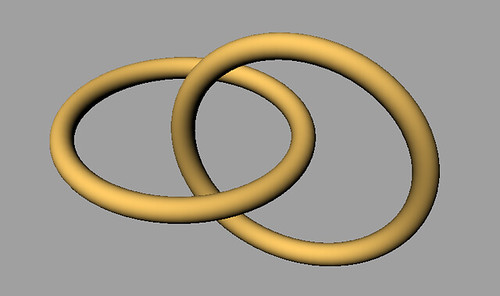# Knots, Topology and DNAAlexander the Great and the Gordian Knot

Untying a knot can be hard. Alexander the Great knew that very well when he decided to cut the Gordian Knot. However, it is worth pointing out that despite his brute force algorithm to untie the knot there was a substantial amount of thinking behind it. Besides, that is why he is not just Alexander but Alexander the Great. Knot theory is the study of embeddings of topological spaces in other topological spaces. Classical knot theory is concerned with the ways in which a circle or a disjoint union of  circles can be embedded in$R^3$. For the rest of this post, we will call a knot a closed continuous curve that does not intersect itself anywhere. The simplest knot we all know is the circle and the next simplest one is the trefoil, which is shown in the next figure.

The places where the knot crosses itself are called crossings and the crossing number$c(K)$ of the knot$K$ is the number of such places. Having two knots we can define the composition of them as a new knot by deleting a small arc from each knot and connecting the four points with two new arcs. A picture always helps:

Reidemeister shows that all knot deformations can be reduced to three types of moves which are known today as Reidemeister moves. These are shown in the next figure. Notice the double arrows, which mean that we can go in either direction. For instance we can either add a twist or we can remove one if it is already there.

More formally, the main result of Reidemeister is the next theorem.

Reidemeister’s Theorem:  Two links can be continuously deformed into each other iff any diagram of one can be transformed into a diagram of the other by a sequence of Reidemeister moves.

Before we discuss the linking number, which is an important concept, we need to define a link. We shall call a link the union of a finite number of disjoint knots in three dimensional space. Two examples are shown in the figures below. The first one is known as the Hopf link and the second one -I guess- is known to everyone.Olympic GamesConsider two components$K_1,K_2$ in a link$L$ and choose an orientation in each component. Then at each crossing we count either 1 or -1 (in the xor sense) depending on the direction in which the tangent vector to the top must be rotated to coincide with the tangent vector to the bottom.  If the rotation happens clockwise then we count -1, otherwise +1. The linking number between the two knots is defined by doing this operation over all crossings, i.e.,$L(K_1,K_2) = \frac{1}{2} \sum_{p \in \alpha \cap \beta} sign(p)$ where$\alpha,\beta$ are the set of crossings of the two knots respectively.  This number has  several important properties. For instance, it remains unchanged if either of the curves is deformed continuously.  Other important concepts that the reader should be aware of, but we are not going to define here are the writhing number, tangles and knot polynomials. A good place to begin with, as always, is Wikipedia.

For the rest of the post, I would like to discuss an important application of knot theory in biology. Crick and Watson in a scientific breakthrough discovered DNA. This video shows the structure of DNA for those not familiar with it.

Geneticists have discovered that DNA can form knots and links! A mathematical model for a DNA molecule is usually a thin, long, narrow(oriented) twisted ribbon.Twisted ribbon

Supercoiling represents the sum of a twist and a writhe. These typically occur when the linking number changes. For instance, it is known that if we reduce the linking number of a double-strand DNA molecule, then the effect is to cause the DNA molecule to twist and coil. White’s formula expresses the relation between the twist, writhe and supercoiling.

The following video visualizes supercoiling!

The concepts we discussed above as well as the ones we did not discuss get a concrete meaning in the context of DNA supercoiling. Specifically, according to our main source  the following hold:

• Linking number: It is the number of times that one DNA strand  crosses about the other when the DNA is made to lie flat on the table.
• Twisting:  It is the number of helical turns in the DNA.
• Writhing: It is a measure of coiling, bending or non-planarity

Knot theory and more generally topology and geometry already play a key role in understanding the unknotting of DNA and therefore advances in any of these fields is likely to lead to important insights into the code of life.

Sources

 Mathematics of Bioinformatics, Chapter 4 He,Petoukhov

 The combinatorial revolution in knot theory, Sam Nelson (Article in Notices of the American Mathematical Society, Vol. 58,No. 11)